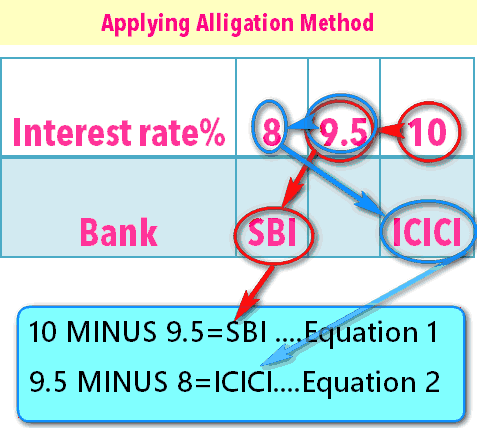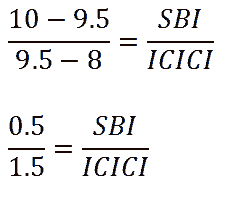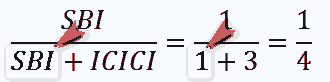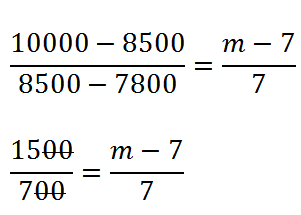Consider these four questions

1. Dr.Haathi has taken some loan from State bank of India at 8% interest rate per annum.He has also borrowed some money from ICICI at 10% interest.The total loan amount is Rs.10,000. He pays total Rs.950 as interest rate at the end of first year.How much money did he borrow from the State bank of India?
2. Jethalal purchased some mobile phone memory cards for Rs.1000. He sold memory cards worth rupees worth Rs.400 at 10% profit.At what percent profit should he sell the remaining memory cards, to gain overall profit of 20%?
3. Roshan Singh Sodhi is the owner of a big hotel. The average salary of all employees is Rs.8500. The average salary of 7 cooks is Rs.10,000.If the average salary of remaining employees, is Rs. 7800. Find the total number of people employed by Roshan Singh Sodhi.
4. Bhide master bought a set of laptop and printer for Rs.20000.He sold the laptop for 20% profit and printer for 10% loss.Overall, he made 2% profit. Find the cost price of laptop.
• Superficially, ^these questions seem different from one another. But if you pay close attention, these are all problems of weighted average.
• We can quickly solve them using the allegation (wine and water mixture) method.

# Case #1: Loan taken from two banks

Dr.Haathi has taken some loan from SBI  at 8% interest and  ICICI at 10% interest.
The total loan amount is Rs.10,000. He pays total Rs.950 as interest rate at the end of first year. How much money did he borrow from the SBI

The question is mentioning two interest rates: 8% and 10%
the effective interest rate, that Dr Haathi has to pay, will be in between these two numbers 8 and 10.
The question also says that he paid Rs.950 as interest rate.
So in terms of percentage he has paid
(950/10000) x 100 =9.5% interest rate (overall).

Arrange these numbers in to ascending (increasing) order

 Interest rate% 8 9.5 10 Bank SBI Overall ICICI

Now apply the “visual move” of Wine and water mixture10-9.5=SBI …eq 1
Similarly
9.5-8=ICICI….eq 2 (donot make mistake of 8-9.5 because we donot want negative difference)

Divide eq. 1 with eq 2If you simplify this further, you get

SBI / ICICI =1/3

Apply Componendo at bottomBut we know that SBI + ICICI=Rs.10,000 that Dr.Haathi has borrowed!

Plug in the value
SBI /10000 = ¼
Therefore, SBI =10,000/4=Rs.2500
It means Dr.Haathi has borrowed Rs.2500 from SBI  Bank.

## Verifying the answer and concept

 Amount borrowed Interest rate SBI 2500 8% of 2500=200 ICICI 10k-2500=7500 10% of 7500=750 Total 8500 200+750=Rs.950 paid in interest = matches the question Description!0

# Case: Profit on Single commodity

• Jethalal purchased some mobile phone memory cards for Rs.1000.
• He sold memory cards worth rupees worth Rs.400 at 10% profit.
• At what percent profit should he sell the remaining memory cards, to gain overall profit of 20%?

If he wants to have overall (average) profit of 20% then he must sell the remaining cards at a profit higher than 20%
The question says he sold cards worth Rs.400 (cost price). Meaning the cost price of remaining cards is 1000-400=Rs.600
Draw a table.

 Profit 10% 20% M% Overall Cards worth (cost price) 400 1000-400=600

Apply the visual move of wine-water mixture
M-20=400….eq1
20-10=600….eq2 (donot make mistake of 10-20 because we don’t want negative)
Now Divide equation 1 with equation 2Meaning, if Jethalal wants to make overall profit of 20%, he must sell the remaining memory cards @26 (2/3)% interest rate.

 Cards already sold @10% interest 10% x 400=Rs.40 Remaining cards sell @26 (2/3) 26 (2/3)% x 600=Rs.160 Total profit Rs 40 +160 =200. And 200 is 20% of Rs.1000 so it matches our question condition.

# Case: No. of Employees based on Avg Salary

• Roshan Singh Sodhi is the owner of a big hotel.
• The average salary of all employees is Rs.8500.
• The average salary of 7 cooks is Rs.10,000.
• If the average salary of remaining employees, is Rs. 7800. Find the total number of people employed by Sodhi.

The question is talking about three sets of salaries
7800 > 8500 (average) >10000
Suppose total number of employees =m.
And we already know there are 7 cooks.
So remaining employees = total minus 7 = m-7.

Draw the table.

 Salary (Rs.) 7800 8500 10000 Overall Employees (total =m) m-7 7 cooks

Apply the visual move
10,000 minus 8500 = (m-7)…eq1
8500 minus 7800=7…eq2
Now Divide equation 1 with equation 2When you simplify this further, you get m=22
Therefore, Total number of employees is 22.

## Verification

 Number Avg. salary Total cost to Sodhi Cooks 7 10000 7 x 10k=70k Others 15 7800 1,17,000 Total 15+7=22 — 70k+1,17,000=1,87,000

Avg salary of all employees
=(Total salary paid)/(Total number of employees)
=187000/22
=Rs.8500 = matches the question condition.

# Case: Profit on Two commodities

• Bhide master bought a set of laptop and printer for Rs.20000.
• He sold the laptop for 20% profit and printer for 10% loss.
• Overall, he made 2% profit. Find the cost price of laptop.

## Approach

We’ll take 10% loss =-10%
Now the question is talking about three rates : -10% , +2% (avg) and +20%

 Profit/loss -10% 2% 20% Overall Cost price Printer Laptop

That’s it. Just apply the visual move BUT with care!
20 minus 2=printer…eq 1

Be Careful while approaching second equation
2 minus (-10)=laptop
2-(-10)=laptop
Hence 2+10=laptop …eq2

Rest is easy. Just divide eq.1 with eq.2When you simplify it further, you get the ratio
printer/laptop=3/2
we’re interested in the Cost price of laptop. So we’ll apply componendo @topBut question already said hat total investment (cost price) of printer +laptop =Rs.20000
Plug in the value
20000/laptop=5/2
Therefore cost price of laptop=(2/5) x 20000=Rs.8000

## Verification

 Cost price Profit/loss Laptop 8,000 20% profit on 8k=1600 Printer 20,000-8000=12,000 10% loss on 12k=Minus 1200 Total 20,00 1600-1200=+400 Rs. as profit

So overall he received Rs.400 profit on the investment of Rs.20,000
=(400/20000) x 100
=2% profit= matches the condition given in question statement.

# Summary

• Weighted average method works when we’ve 3 rates (including one average) and 2 quantities.
• First arrange the rates in ascending order. Then draw the table with corresponding rates and quantities.
• Apply “visual” move and get the answer.

# Mock Questions

Case: Journalist Popatlal took some loan from Matkaa-King Mohanlal @36% interest rate and some loan from Taarak Mehta @10% interest rate. Total money borrowed Rs.6000. At the end of first year, Popat paid total interest of Rs.912. Answer following questions

1. Total amount borrowed
2. Money borrowed from Mehta
3. Money borrowed from Mohan
4. Effective interest rate on total borrowing?

Case: Jethalal bought a chair+table set @Rs.6000. Later he sold Chair @10% loss and table @10 profit. Overall he gained Rs.100. Answer following questions

1. Cost price of Chair
2. Cost price of Table
3. Selling price of Chair
4. Selling Price of Table

Case: In Tappu’s class, the average weight of boys and girls is 77.5kg, 70kg respectively. Overall average weight of all students is 74 kg.

1. Find Ratio of Boys: girls
2. If total number of students is 30, how many boys study in Tappu’s class?
3. At present, total number of students is 60. If school administration wants to have equal number of boys and girls in this class, how many new girls should be admitted?

Case: In a state, total number of Desi-liquor dens and Land mafias is 100. They pay total Rs.1650 crores bribe to home minister every year. Average bribe paid by each Desi-liquor den and land mafia is 15 crores and 20 crores respectively.

1. Find total number of land mafias in this state
2. Final total bribe paid by these land mafias
3. Owing to media-pressure, the home minister orders police to close down some of the desi-liquor dens. Now his total bribe income declined by 20%. What is the new ratio of liquor den: land mafias?

Case: A parking lot has only cars and bikes. Average number of tires per vehicle is 2.9. There are 55 bikes in this parking plot.

1. Find total number of cars
2. If 5 cars are added and 5 bikes are removed, what’ll be the new average number of tires per vehicle?

## Popat in debt

1. Total borrowed Rs. 6000
2. 4800 from Mehta
3. 1200 from Mohanlal.
4. 15.2% interest rate (overall)

## Jetha

1. Cost price Chair =2500
2. CP Table= 3500.
3. Selling price of Table (+10% profit)=1.1 x 3500=3850
4. Selling price of Chair (-10% loss)=0.9 x2500=2250

## School

1. Boys/Girls=8/7
2. 16 boys and 14 girls.
3. 4 new girls need to be admitted.

## Home minister

1. Avg. annual bribe is 1650/100=16.5 Now the rates are 15>>16.5>>20. Ratio of liquor-dens:mafia=7:3. Since total are 100, number of Mafia=3/10 x 100=30.
2. 30 mafia x avg 20 crores=600 crores paid by them.
3. 20% of 1650=330. And 330/15=22 liquor dens were closed down. So new ratio is (70-22):30=48:30=8/5

## Parking lot

1. Number of cars=45.
2. New avg. no. of tires per vehicle=3

For the Archive of all [Aptitude] Articles, visit Mrunal.org/aptitude